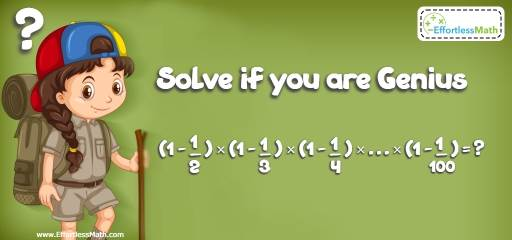# Ratio, Proportion and Percentages Puzzle – Critical Thinking 7

This kind of math puzzle is an educational activity for practicing children's math skills. It's also helpful for adults to have fun and challenge their brains!## Challenge:

$$(1-\frac{1}{2}) × (1 -\frac{1}{2}) × (1-\frac{1}{4} ) × … × (1-\frac{1}{100} ) =$$ ?

## The Absolute Best Book to challenge your Smart Student!

The correct answer is $$\frac{1}{100}$$.

First, simplify the expression.
$$(1-\frac{1}{2}) × (1 -\frac{1}{2}) × (1-\frac{1}{4} ) × … × (1-\frac{1}{100} ) =$$ ?
$$(\frac{1}{2} ) × ( \frac{2}{3}) × ( \frac{3}{4}) × … × ( \frac{99}{100}) =$$
Remove the same numbers from both numerator and denominator. For example, cancel out $$2, 3, 4,$$ … from both numerator and denominator. You’ll get:$$\frac{1}{100}$$

The Absolute Best Books to Ace Algebra

### What people say about "Ratio, Proportion and Percentages Puzzle – Critical Thinking 7 - Effortless Math: We Help Students Learn to LOVE Mathematics"?

No one replied yet.

X
45% OFF

Limited time only!

Save Over 45%

SAVE $40 It was$89.99 now it is \$49.99